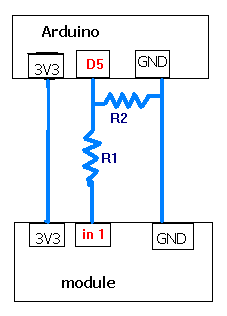# Control all 3.3V devices with 5V Arduino ?

Hello,

there are many 3.3V modules for other microcontrollers out there (e.g. there are Arduinos with 3.3V supply voltage).

To work with them, I though of this simple construction:with:

``````R[sub]2[/sub] = 2*R[sub]1[/sub]
``````

Then we have

``````3.3V = 5V * R[sub]2[/sub]/(R[sub]1[/sub]+R[sub]2[/sub])
``````
``````3.3V = 5V * 2*R[sub]1[/sub]/(R[sub]1[/sub]+2*R[sub]1[/sub])
``````
``````3.3V = 5V * 2*R[sub]1[/sub]/(3*R[sub]1[/sub])
``````

`3.3V = 5V * 2/3`, check OK

So I can use for example: `R[sub]1[/sub] = 220?` `R[sub]2[/sub] = 220?+220? (series switch)`

Is this thinking correct? Or is there any mistake. Then it is very easy because you only need one type of resistor and double it by doing series .

Thank you very much. Then I know whether I can buy all the 3.3V modules :)

~

Well a typical voltage divider solution to change a 5vdc output signal to a 3.3vdc input signal would have D5 digital output pin wire to one end of R1, the other end of R1 to R2, the other end of R2 to ground. Then a wire from the junction of R1 and R2 to the 3.3 input pin. So just calculate the resistance ratios needed to convert 5v to 3.3 volt.

Lefty

Aaaahrg, I mixed the order of the resitors!! This is corrected:My question is whether it is really that simple?!

karlok: Aaaahrg, I mixed the order of the resitors!! This is corrected:My question is whether it is really that simple?!

Yes it is. You do however have to size the resistors such that total series resistance of R1 + R2 does not draw more current then the 5vdc digital output pin can provide, typical sized to draw just a few milliamps is fine. You also have to be sure the input device doesn't draw much current at all as that will upset the voltage division factor, however most digital inputs draw very little current, some ua at most, so can be ignored in the resistor calculations. If you want to eliminate all calculations just use a small trimmer pot (say 5k or 10k ohms) wired between D5 and ground, with the wiper terminal adjusted to 3.3vdc and wired to the 3.3v input pin.

Lefty

Unfortunately, I have no potentiometer, but resistors: 220? 1K? 10K? each 10 times, not more. I think 40mA is max current drawn per pin

So I have an input device whose current drawn can be ignored, 2*220?+1*220?=3*220?=660? (=R1+R2). I = 5V/660? = 7.5mA

Is calculation correct? However, that means I could also use 10K? because current drawn gets less with increasing resistor value (?)

You should always read the data sheet for these modules, some will take 5v because the chips is able to or the module has an inbuilt voltage divider.

The modules 3.3v high outputs can go direct to the arduino as the digital inputs see anything over 3v as high.

Mark

holmes4:
You should always read the data sheet for these modules, some will take 5v because the chips is able to or the module has an inbuilt voltage divider.

The modules 3.3v high outputs can go direct to the arduino as the digital inputs see anything over 3v as high.

Yeah, I knew that alreadyThe question is using those 3.3V modules not having an intern voltage divider and as output.

karlok:
Unfortunately, I have no potentiometer, but resistors:
220?
1K?
10K?
each 10 times, not more.
I think 40mA is max current drawn per pin

So I have an input device whose current drawn can be ignored, 2220?+1220?=3*220?=660? (=R1+R2).
I = 5V/660? = 7.5mA

Is calculation correct?
However, that means I could also use 10K? because current drawn gets less with increasing resistor value (?)

So use 3 1k resistors. One 1 k resistor will represent R1, 2 1k resistors wired in series will represent R2. Junction of R1 and R2 will be 3.3vdc if R1 driven with 5vdc and divider current will be about 1.6ma.

Lefty

Ok, Could I also use 10K resistors? That would minimize the current drawn. And, thank you very much for the explanation.

karlok: Ok, Could I also use 10K resistors? That would minimize the current drawn. And, thank you very much for the explanation.

You could try, but I would feel better running at the 1.6 ma rate, rather then at 160 uamp rate.

Lefty

Ok, that is fine! And thank you very much for the help. This is a very easy way to work with 3.3V modules :)